# Gradient Boosted Trees: Model understanding

For an end-to-end walkthrough of training a Gradient Boosting model check out the boosted trees tutorial. In this tutorial you will:

• Learn how to interpret a Boosted Trees model both locally and globally
• Gain intution for how a Boosted Trees model fits a dataset

## How to interpret Boosted Trees models both locally and globally

Local interpretability refers to an understanding of a model’s predictions at the individual example level, while global interpretability refers to an understanding of the model as a whole. Such techniques can help machine learning (ML) practitioners detect bias and bugs during the model development stage.

For local interpretability, you will learn how to create and visualize per-instance contributions. To distinguish this from feature importances, we refer to these values as directional feature contributions (DFCs).

For global interpretability you will retrieve and visualize gain-based feature importances, permutation feature importances and also show aggregated DFCs.

You will be using the titanic dataset, where the (rather morbid) goal is to predict passenger survival, given characteristics such as gender, age, class, etc.

import numpy as np
import pandas as pd
from IPython.display import clear_output

y_train = dftrain.pop('survived')
y_eval = dfeval.pop('survived')

import tensorflow as tf
tf.random.set_seed(123)

TensorFlow 2.x selected.



For a description of the features, please review the prior tutorial.

## Create feature columns, input_fn, and the train the estimator

### Preprocess the data

Create the feature columns, using the original numeric columns as is and one-hot-encoding categorical variables.

fc = tf.feature_column
CATEGORICAL_COLUMNS = ['sex', 'n_siblings_spouses', 'parch', 'class', 'deck',
'embark_town', 'alone']
NUMERIC_COLUMNS = ['age', 'fare']

def one_hot_cat_column(feature_name, vocab):
return fc.indicator_column(
fc.categorical_column_with_vocabulary_list(feature_name,
vocab))
feature_columns = []
for feature_name in CATEGORICAL_COLUMNS:
# Need to one-hot encode categorical features.
vocabulary = dftrain[feature_name].unique()
feature_columns.append(one_hot_cat_column(feature_name, vocabulary))

for feature_name in NUMERIC_COLUMNS:
feature_columns.append(fc.numeric_column(feature_name,
dtype=tf.float32))


### Build the input pipeline

Create the input functions using the from_tensor_slices method in the tf.data API to read in data directly from Pandas.

# Use entire batch since this is such a small dataset.
NUM_EXAMPLES = len(y_train)

def make_input_fn(X, y, n_epochs=None, shuffle=True):
def input_fn():
dataset = tf.data.Dataset.from_tensor_slices((X.to_dict(orient='list'), y))
if shuffle:
dataset = dataset.shuffle(NUM_EXAMPLES)
# For training, cycle thru dataset as many times as need (n_epochs=None).
dataset = (dataset
.repeat(n_epochs)
.batch(NUM_EXAMPLES))
return dataset
return input_fn

# Training and evaluation input functions.
train_input_fn = make_input_fn(dftrain, y_train)
eval_input_fn = make_input_fn(dfeval, y_eval, shuffle=False, n_epochs=1)


### Train the model

params = {
'n_trees': 50,
'max_depth': 3,
'n_batches_per_layer': 1,
# You must enable center_bias = True to get DFCs. This will force the model to
# make an initial prediction before using any features (e.g. use the mean of
# the training labels for regression or log odds for classification when
# using cross entropy loss).
'center_bias': True
}

est = tf.estimator.BoostedTreesClassifier(feature_columns, **params)
# Train model.
est.train(train_input_fn, max_steps=100)

# Evaluation.
results = est.evaluate(eval_input_fn)
clear_output()
pd.Series(results).to_frame()


For performance reasons, when your data fits in memory, we recommend use the boosted_trees_classifier_train_in_memory function. However if training time is not of a concern or if you have a very large dataset and want to do distributed training, use the tf.estimator.BoostedTrees API shown above.

When using this method, you should not batch your input data, as the method operates on the entire dataset.

in_memory_params = dict(params)
in_memory_params['n_batches_per_layer'] = 1
# In-memory input_fn does not use batching.
def make_inmemory_train_input_fn(X, y):
y = np.expand_dims(y, axis=1)
def input_fn():
return dict(X), y
return input_fn
train_input_fn = make_inmemory_train_input_fn(dftrain, y_train)

# Train the model.
est = tf.estimator.BoostedTreesClassifier(
feature_columns,
train_in_memory=True,
**in_memory_params)

est.train(train_input_fn)
print(est.evaluate(eval_input_fn))

INFO:tensorflow:Using default config.
WARNING:tensorflow:Using temporary folder as model directory: /tmp/tmpec8e696f
INFO:tensorflow:Using config: {'_model_dir': '/tmp/tmpec8e696f', '_tf_random_seed': None, '_save_summary_steps': 100, '_save_checkpoints_steps': None, '_save_checkpoints_secs': 600, '_session_config': allow_soft_placement: true
graph_options {
rewrite_options {
meta_optimizer_iterations: ONE
}
}
, '_keep_checkpoint_max': 5, '_keep_checkpoint_every_n_hours': 10000, '_log_step_count_steps': 100, '_train_distribute': None, '_device_fn': None, '_protocol': None, '_eval_distribute': None, '_experimental_distribute': None, '_experimental_max_worker_delay_secs': None, '_session_creation_timeout_secs': 7200, '_service': None, '_cluster_spec': ClusterSpec({}), '_task_type': 'worker', '_task_id': 0, '_global_id_in_cluster': 0, '_master': '', '_evaluation_master': '', '_is_chief': True, '_num_ps_replicas': 0, '_num_worker_replicas': 1}
INFO:tensorflow:Calling model_fn.
INFO:tensorflow:Done calling model_fn.
INFO:tensorflow:Create CheckpointSaverHook.
WARNING:tensorflow:Issue encountered when serializing resources.
Type is unsupported, or the types of the items don't match field type in CollectionDef. Note this is a warning and probably safe to ignore.
'_Resource' object has no attribute 'name'
INFO:tensorflow:Graph was finalized.
INFO:tensorflow:Running local_init_op.
INFO:tensorflow:Done running local_init_op.
WARNING:tensorflow:Issue encountered when serializing resources.
Type is unsupported, or the types of the items don't match field type in CollectionDef. Note this is a warning and probably safe to ignore.
'_Resource' object has no attribute 'name'
INFO:tensorflow:Saving checkpoints for 0 into /tmp/tmpec8e696f/model.ckpt.
WARNING:tensorflow:Issue encountered when serializing resources.
Type is unsupported, or the types of the items don't match field type in CollectionDef. Note this is a warning and probably safe to ignore.
'_Resource' object has no attribute 'name'
INFO:tensorflow:loss = 0.6931472, step = 0
WARNING:tensorflow:It seems that global step (tf.train.get_global_step) has not been increased. Current value (could be stable): 0 vs previous value: 0. You could increase the global step by passing tf.train.get_global_step() to Optimizer.apply_gradients or Optimizer.minimize.
INFO:tensorflow:global_step/sec: 80.2732
INFO:tensorflow:loss = 0.34654337, step = 99 (1.249 sec)
INFO:tensorflow:Saving checkpoints for 153 into /tmp/tmpec8e696f/model.ckpt.
WARNING:tensorflow:Issue encountered when serializing resources.
Type is unsupported, or the types of the items don't match field type in CollectionDef. Note this is a warning and probably safe to ignore.
'_Resource' object has no attribute 'name'
INFO:tensorflow:Loss for final step: 0.31796658.
INFO:tensorflow:Calling model_fn.
WARNING:tensorflow:Trapezoidal rule is known to produce incorrect PR-AUCs; please switch to "careful_interpolation" instead.
WARNING:tensorflow:Trapezoidal rule is known to produce incorrect PR-AUCs; please switch to "careful_interpolation" instead.
INFO:tensorflow:Done calling model_fn.
INFO:tensorflow:Starting evaluation at 2020-03-09T21:21:14Z
INFO:tensorflow:Graph was finalized.
INFO:tensorflow:Restoring parameters from /tmp/tmpec8e696f/model.ckpt-153
INFO:tensorflow:Running local_init_op.
INFO:tensorflow:Done running local_init_op.
INFO:tensorflow:Inference Time : 0.55945s
INFO:tensorflow:Finished evaluation at 2020-03-09-21:21:15
INFO:tensorflow:Saving dict for global step 153: accuracy = 0.8030303, accuracy_baseline = 0.625, auc = 0.8679216, auc_precision_recall = 0.8527449, average_loss = 0.4203342, global_step = 153, label/mean = 0.375, loss = 0.4203342, precision = 0.7473684, prediction/mean = 0.38673538, recall = 0.7171717
WARNING:tensorflow:Issue encountered when serializing resources.
Type is unsupported, or the types of the items don't match field type in CollectionDef. Note this is a warning and probably safe to ignore.
'_Resource' object has no attribute 'name'
INFO:tensorflow:Saving 'checkpoint_path' summary for global step 153: /tmp/tmpec8e696f/model.ckpt-153
{'accuracy': 0.8030303, 'accuracy_baseline': 0.625, 'auc': 0.8679216, 'auc_precision_recall': 0.8527449, 'average_loss': 0.4203342, 'label/mean': 0.375, 'loss': 0.4203342, 'precision': 0.7473684, 'prediction/mean': 0.38673538, 'recall': 0.7171717, 'global_step': 153}



## Model interpretation and plotting

import matplotlib.pyplot as plt
import seaborn as sns
sns_colors = sns.color_palette('colorblind')


## Local interpretability

Next you will output the directional feature contributions (DFCs) to explain individual predictions using the approach outlined in Palczewska et al and by Saabas in Interpreting Random Forests (this method is also available in scikit-learn for Random Forests in the treeinterpreter package). The DFCs are generated with:

pred_dicts = list(est.experimental_predict_with_explanations(pred_input_fn))

(Note: The method is named experimental as we may modify the API before dropping the experimental prefix.)

pred_dicts = list(est.experimental_predict_with_explanations(eval_input_fn))

INFO:tensorflow:Using config: {'_model_dir': '/tmp/tmpec8e696f', '_tf_random_seed': None, '_save_summary_steps': 100, '_save_checkpoints_steps': None, '_save_checkpoints_secs': 600, '_session_config': allow_soft_placement: true
graph_options {
rewrite_options {
meta_optimizer_iterations: ONE
}
}
, '_keep_checkpoint_max': 5, '_keep_checkpoint_every_n_hours': 10000, '_log_step_count_steps': 100, '_train_distribute': None, '_device_fn': None, '_protocol': None, '_eval_distribute': None, '_experimental_distribute': None, '_experimental_max_worker_delay_secs': None, '_session_creation_timeout_secs': 7200, '_service': None, '_cluster_spec': ClusterSpec({}), '_task_type': 'worker', '_task_id': 0, '_global_id_in_cluster': 0, '_master': '', '_evaluation_master': '', '_is_chief': True, '_num_ps_replicas': 0, '_num_worker_replicas': 1}
INFO:tensorflow:Calling model_fn.
INFO:tensorflow:Done calling model_fn.
INFO:tensorflow:Graph was finalized.
INFO:tensorflow:Restoring parameters from /tmp/tmpec8e696f/model.ckpt-153
INFO:tensorflow:Running local_init_op.
INFO:tensorflow:Done running local_init_op.


# Create DFC Pandas dataframe.
labels = y_eval.values
probs = pd.Series([pred['probabilities'] for pred in pred_dicts])
df_dfc = pd.DataFrame([pred['dfc'] for pred in pred_dicts])
df_dfc.describe().T


A nice property of DFCs is that the sum of the contributions + the bias is equal to the prediction for a given example.

# Sum of DFCs + bias == probabality.
bias = pred_dicts['bias']
dfc_prob = df_dfc.sum(axis=1) + bias
np.testing.assert_almost_equal(dfc_prob.values,
probs.values)


Plot DFCs for an individual passenger. Let's make the plot nice by color coding based on the contributions' directionality and add the feature values on figure.

# Boilerplate code for plotting :)
def _get_color(value):
"""To make positive DFCs plot green, negative DFCs plot red."""
green, red = sns.color_palette()[2:4]
if value >= 0: return green
return red

"""Display feature's values on left of plot."""
x_coord = ax.get_xlim()
OFFSET = 0.15
for y_coord, (feat_name, feat_val) in enumerate(feature_values.items()):
t = plt.text(x_coord, y_coord - OFFSET, '{}'.format(feat_val), size=12)
t.set_bbox(dict(facecolor='white', alpha=0.5))
from matplotlib.font_manager import FontProperties
font = FontProperties()
font.set_weight('bold')
t = plt.text(x_coord, y_coord + 1 - OFFSET, 'feature\nvalue',
fontproperties=font, size=12)

def plot_example(example):
TOP_N = 8 # View top 8 features.
sorted_ix = example.abs().sort_values()[-TOP_N:].index  # Sort by magnitude.
example = example[sorted_ix]
colors = example.map(_get_color).tolist()
ax = example.to_frame().plot(kind='barh',
color=[colors],
legend=None,
alpha=0.75,
figsize=(10,6))
ax.grid(False, axis='y')
ax.set_yticklabels(ax.get_yticklabels(), size=14)

return ax

# Plot results.
ID = 182
example = df_dfc.iloc[ID]  # Choose ith example from evaluation set.
TOP_N = 8  # View top 8 features.
sorted_ix = example.abs().sort_values()[-TOP_N:].index
ax = plot_example(example)
ax.set_title('Feature contributions for example {}\n pred: {:1.2f}; label: {}'.format(ID, probs[ID], labels[ID]))
ax.set_xlabel('Contribution to predicted probability', size=14)
plt.show()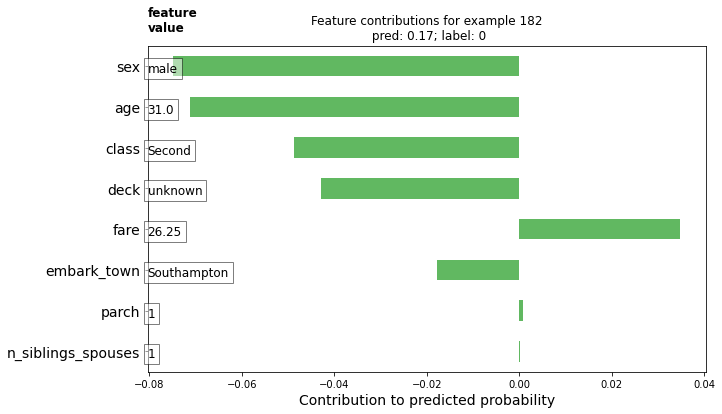The larger magnitude contributions have a larger impact on the model's prediction. Negative contributions indicate the feature value for this given example reduced the model's prediction, while positive values contribute an increase in the prediction.

You can also plot the example's DFCs compare with the entire distribution using a voilin plot.

# Boilerplate plotting code.
def dist_violin_plot(df_dfc, ID):
# Initialize plot.
fig, ax = plt.subplots(1, 1, figsize=(10, 6))

# Create example dataframe.
TOP_N = 8  # View top 8 features.
example = df_dfc.iloc[ID]
ix = example.abs().sort_values()[-TOP_N:].index
example = example[ix]
example_df = example.to_frame(name='dfc')

# Add contributions of entire distribution.
parts=ax.violinplot([df_dfc[w] for w in ix],
vert=False,
showextrema=False,
widths=0.7,
positions=np.arange(len(ix)))
face_color = sns_colors
alpha = 0.15
for pc in parts['bodies']:
pc.set_facecolor(face_color)
pc.set_alpha(alpha)

ax.scatter(example,
np.arange(example.shape),
color=sns.color_palette(),
s=100,
marker="s",
label='contributions for example')

# Legend
# Proxy plot, to show violinplot dist on legend.
ax.plot([0,0], [1,1], label='eval set contributions\ndistributions',
color=face_color, alpha=alpha, linewidth=10)
legend = ax.legend(loc='lower right', shadow=True, fontsize='x-large',
frameon=True)
legend.get_frame().set_facecolor('white')

# Format plot.
ax.set_yticks(np.arange(example.shape))
ax.set_yticklabels(example.index)
ax.grid(False, axis='y')
ax.set_xlabel('Contribution to predicted probability', size=14)


Plot this example.

dist_violin_plot(df_dfc, ID)
plt.title('Feature contributions for example {}\n pred: {:1.2f}; label: {}'.format(ID, probs[ID], labels[ID]))
plt.show()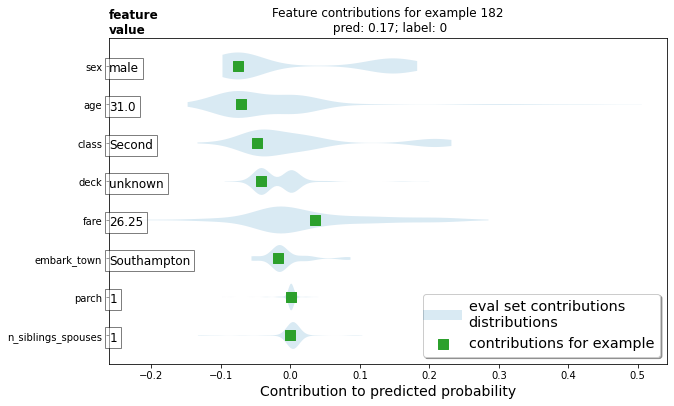Finally, third-party tools, such as LIME and shap, can also help understand individual predictions for a model.

## Global feature importances

Additionally, you might want to understand the model as a whole, rather than studying individual predictions. Below, you will compute and use:

• Gain-based feature importances using est.experimental_feature_importances
• Permutation importances
• Aggregate DFCs using est.experimental_predict_with_explanations

Gain-based feature importances measure the loss change when splitting on a particular feature, while permutation feature importances are computed by evaluating model performance on the evaluation set by shuffling each feature one-by-one and attributing the change in model performance to the shuffled feature.

In general, permutation feature importance are preferred to gain-based feature importance, though both methods can be unreliable in situations where potential predictor variables vary in their scale of measurement or their number of categories and when features are correlated (source). Check out this article for an in-depth overview and great discussion on different feature importance types.

### Gain-based feature importances

Gain-based feature importances are built into the TensorFlow Boosted Trees estimators using est.experimental_feature_importances.

importances = est.experimental_feature_importances(normalize=True)
df_imp = pd.Series(importances)

# Visualize importances.
N = 8
ax = (df_imp.iloc[0:N][::-1]
.plot(kind='barh',
color=sns_colors,
title='Gain feature importances',
figsize=(10, 6)))
ax.grid(False, axis='y')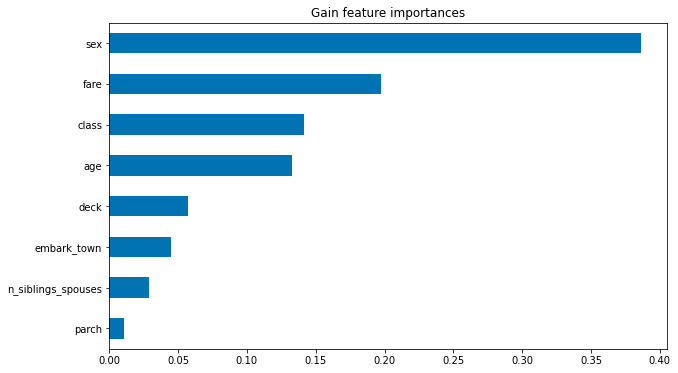### Average absolute DFCs

You can also average the absolute values of DFCs to understand impact at a global level.

# Plot.
dfc_mean = df_dfc.abs().mean()
N = 8
sorted_ix = dfc_mean.abs().sort_values()[-N:].index  # Average and sort by absolute.
ax = dfc_mean[sorted_ix].plot(kind='barh',
color=sns_colors,
title='Mean |directional feature contributions|',
figsize=(10, 6))
ax.grid(False, axis='y')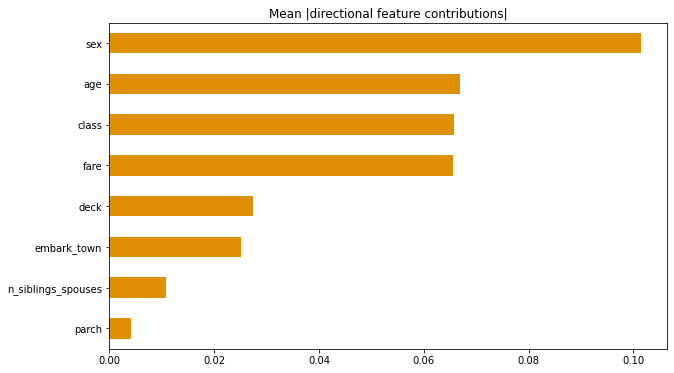You can also see how DFCs vary as a feature value varies.

FEATURE = 'fare'
feature = pd.Series(df_dfc[FEATURE].values, index=dfeval[FEATURE].values).sort_index()
ax = sns.regplot(feature.index.values, feature.values, lowess=True)
ax.set_ylabel('contribution')
ax.set_xlabel(FEATURE)
ax.set_xlim(0, 100)
plt.show()### Permutation feature importance

def permutation_importances(est, X_eval, y_eval, metric, features):
"""Column by column, shuffle values and observe effect on eval set.

source: http://explained.ai/rf-importance/index.html
A similar approach can be done during training. See "Drop-column importance"
in the above article."""
baseline = metric(est, X_eval, y_eval)
imp = []
for col in features:
save = X_eval[col].copy()
X_eval[col] = np.random.permutation(X_eval[col])
m = metric(est, X_eval, y_eval)
X_eval[col] = save
imp.append(baseline - m)
return np.array(imp)

def accuracy_metric(est, X, y):
"""TensorFlow estimator accuracy."""
eval_input_fn = make_input_fn(X,
y=y,
shuffle=False,
n_epochs=1)
return est.evaluate(input_fn=eval_input_fn)['accuracy']
features = CATEGORICAL_COLUMNS + NUMERIC_COLUMNS
importances = permutation_importances(est, dfeval, y_eval, accuracy_metric,
features)
df_imp = pd.Series(importances, index=features)

sorted_ix = df_imp.abs().sort_values().index
ax = df_imp[sorted_ix][-5:].plot(kind='barh', color=sns_colors, figsize=(10, 6))
ax.grid(False, axis='y')
ax.set_title('Permutation feature importance')
plt.show()

INFO:tensorflow:Calling model_fn.
WARNING:tensorflow:Trapezoidal rule is known to produce incorrect PR-AUCs; please switch to "careful_interpolation" instead.
WARNING:tensorflow:Trapezoidal rule is known to produce incorrect PR-AUCs; please switch to "careful_interpolation" instead.
INFO:tensorflow:Done calling model_fn.
INFO:tensorflow:Starting evaluation at 2020-03-09T21:21:18Z
INFO:tensorflow:Graph was finalized.
INFO:tensorflow:Restoring parameters from /tmp/tmpec8e696f/model.ckpt-153
INFO:tensorflow:Running local_init_op.
INFO:tensorflow:Done running local_init_op.
INFO:tensorflow:Inference Time : 0.56113s
INFO:tensorflow:Finished evaluation at 2020-03-09-21:21:18
INFO:tensorflow:Saving dict for global step 153: accuracy = 0.8030303, accuracy_baseline = 0.625, auc = 0.8679216, auc_precision_recall = 0.8527449, average_loss = 0.4203342, global_step = 153, label/mean = 0.375, loss = 0.4203342, precision = 0.7473684, prediction/mean = 0.38673538, recall = 0.7171717
INFO:tensorflow:Saving 'checkpoint_path' summary for global step 153: /tmp/tmpec8e696f/model.ckpt-153
INFO:tensorflow:Calling model_fn.
WARNING:tensorflow:Trapezoidal rule is known to produce incorrect PR-AUCs; please switch to "careful_interpolation" instead.
WARNING:tensorflow:Trapezoidal rule is known to produce incorrect PR-AUCs; please switch to "careful_interpolation" instead.
INFO:tensorflow:Done calling model_fn.
INFO:tensorflow:Starting evaluation at 2020-03-09T21:21:19Z
INFO:tensorflow:Graph was finalized.
INFO:tensorflow:Restoring parameters from /tmp/tmpec8e696f/model.ckpt-153
INFO:tensorflow:Running local_init_op.
INFO:tensorflow:Done running local_init_op.
INFO:tensorflow:Inference Time : 0.57949s
INFO:tensorflow:Finished evaluation at 2020-03-09-21:21:19
INFO:tensorflow:Saving dict for global step 153: accuracy = 0.6060606, accuracy_baseline = 0.625, auc = 0.64355683, auc_precision_recall = 0.5400543, average_loss = 0.74337494, global_step = 153, label/mean = 0.375, loss = 0.74337494, precision = 0.47524753, prediction/mean = 0.39103043, recall = 0.4848485
INFO:tensorflow:Saving 'checkpoint_path' summary for global step 153: /tmp/tmpec8e696f/model.ckpt-153
INFO:tensorflow:Calling model_fn.
WARNING:tensorflow:Trapezoidal rule is known to produce incorrect PR-AUCs; please switch to "careful_interpolation" instead.
WARNING:tensorflow:Trapezoidal rule is known to produce incorrect PR-AUCs; please switch to "careful_interpolation" instead.
INFO:tensorflow:Done calling model_fn.
INFO:tensorflow:Starting evaluation at 2020-03-09T21:21:20Z
INFO:tensorflow:Graph was finalized.
INFO:tensorflow:Restoring parameters from /tmp/tmpec8e696f/model.ckpt-153
INFO:tensorflow:Running local_init_op.
INFO:tensorflow:Done running local_init_op.
INFO:tensorflow:Inference Time : 0.58528s
INFO:tensorflow:Finished evaluation at 2020-03-09-21:21:21
INFO:tensorflow:Saving dict for global step 153: accuracy = 0.7916667, accuracy_baseline = 0.625, auc = 0.8624732, auc_precision_recall = 0.8392693, average_loss = 0.43363357, global_step = 153, label/mean = 0.375, loss = 0.43363357, precision = 0.7244898, prediction/mean = 0.38975066, recall = 0.7171717
INFO:tensorflow:Saving 'checkpoint_path' summary for global step 153: /tmp/tmpec8e696f/model.ckpt-153
INFO:tensorflow:Calling model_fn.
WARNING:tensorflow:Trapezoidal rule is known to produce incorrect PR-AUCs; please switch to "careful_interpolation" instead.
WARNING:tensorflow:Trapezoidal rule is known to produce incorrect PR-AUCs; please switch to "careful_interpolation" instead.
INFO:tensorflow:Done calling model_fn.
INFO:tensorflow:Starting evaluation at 2020-03-09T21:21:21Z
INFO:tensorflow:Graph was finalized.
INFO:tensorflow:Restoring parameters from /tmp/tmpec8e696f/model.ckpt-153
INFO:tensorflow:Running local_init_op.
INFO:tensorflow:Done running local_init_op.
INFO:tensorflow:Inference Time : 0.55600s
INFO:tensorflow:Finished evaluation at 2020-03-09-21:21:22
INFO:tensorflow:Saving dict for global step 153: accuracy = 0.8068182, accuracy_baseline = 0.625, auc = 0.8674931, auc_precision_recall = 0.85280114, average_loss = 0.4206087, global_step = 153, label/mean = 0.375, loss = 0.4206087, precision = 0.75, prediction/mean = 0.38792592, recall = 0.72727275
INFO:tensorflow:Saving 'checkpoint_path' summary for global step 153: /tmp/tmpec8e696f/model.ckpt-153
INFO:tensorflow:Calling model_fn.
WARNING:tensorflow:Trapezoidal rule is known to produce incorrect PR-AUCs; please switch to "careful_interpolation" instead.
WARNING:tensorflow:Trapezoidal rule is known to produce incorrect PR-AUCs; please switch to "careful_interpolation" instead.
INFO:tensorflow:Done calling model_fn.
INFO:tensorflow:Starting evaluation at 2020-03-09T21:21:22Z
INFO:tensorflow:Graph was finalized.
INFO:tensorflow:Restoring parameters from /tmp/tmpec8e696f/model.ckpt-153
INFO:tensorflow:Running local_init_op.
INFO:tensorflow:Done running local_init_op.
INFO:tensorflow:Inference Time : 0.54454s
INFO:tensorflow:Finished evaluation at 2020-03-09-21:21:23
INFO:tensorflow:Saving dict for global step 153: accuracy = 0.72727275, accuracy_baseline = 0.625, auc = 0.76737064, auc_precision_recall = 0.62659556, average_loss = 0.6019534, global_step = 153, label/mean = 0.375, loss = 0.6019534, precision = 0.6626506, prediction/mean = 0.3688063, recall = 0.5555556
INFO:tensorflow:Saving 'checkpoint_path' summary for global step 153: /tmp/tmpec8e696f/model.ckpt-153
INFO:tensorflow:Calling model_fn.
WARNING:tensorflow:Trapezoidal rule is known to produce incorrect PR-AUCs; please switch to "careful_interpolation" instead.
WARNING:tensorflow:Trapezoidal rule is known to produce incorrect PR-AUCs; please switch to "careful_interpolation" instead.
INFO:tensorflow:Done calling model_fn.
INFO:tensorflow:Starting evaluation at 2020-03-09T21:21:24Z
INFO:tensorflow:Graph was finalized.
INFO:tensorflow:Restoring parameters from /tmp/tmpec8e696f/model.ckpt-153
INFO:tensorflow:Running local_init_op.
INFO:tensorflow:Done running local_init_op.
INFO:tensorflow:Inference Time : 0.53149s
INFO:tensorflow:Finished evaluation at 2020-03-09-21:21:24
INFO:tensorflow:Saving dict for global step 153: accuracy = 0.7878788, accuracy_baseline = 0.625, auc = 0.8389348, auc_precision_recall = 0.8278463, average_loss = 0.45054114, global_step = 153, label/mean = 0.375, loss = 0.45054114, precision = 0.7263158, prediction/mean = 0.3912348, recall = 0.6969697
INFO:tensorflow:Saving 'checkpoint_path' summary for global step 153: /tmp/tmpec8e696f/model.ckpt-153
INFO:tensorflow:Calling model_fn.
WARNING:tensorflow:Trapezoidal rule is known to produce incorrect PR-AUCs; please switch to "careful_interpolation" instead.
WARNING:tensorflow:Trapezoidal rule is known to produce incorrect PR-AUCs; please switch to "careful_interpolation" instead.
INFO:tensorflow:Done calling model_fn.
INFO:tensorflow:Starting evaluation at 2020-03-09T21:21:25Z
INFO:tensorflow:Graph was finalized.
INFO:tensorflow:Restoring parameters from /tmp/tmpec8e696f/model.ckpt-153
INFO:tensorflow:Running local_init_op.
INFO:tensorflow:Done running local_init_op.
INFO:tensorflow:Inference Time : 0.54399s
INFO:tensorflow:Finished evaluation at 2020-03-09-21:21:25
INFO:tensorflow:Saving dict for global step 153: accuracy = 0.8030303, accuracy_baseline = 0.625, auc = 0.862565, auc_precision_recall = 0.84412414, average_loss = 0.42553493, global_step = 153, label/mean = 0.375, loss = 0.42553493, precision = 0.75268817, prediction/mean = 0.37500647, recall = 0.7070707
INFO:tensorflow:Saving 'checkpoint_path' summary for global step 153: /tmp/tmpec8e696f/model.ckpt-153
INFO:tensorflow:Calling model_fn.
WARNING:tensorflow:Trapezoidal rule is known to produce incorrect PR-AUCs; please switch to "careful_interpolation" instead.
WARNING:tensorflow:Trapezoidal rule is known to produce incorrect PR-AUCs; please switch to "careful_interpolation" instead.
INFO:tensorflow:Done calling model_fn.
INFO:tensorflow:Starting evaluation at 2020-03-09T21:21:26Z
INFO:tensorflow:Graph was finalized.
INFO:tensorflow:Restoring parameters from /tmp/tmpec8e696f/model.ckpt-153
INFO:tensorflow:Running local_init_op.
INFO:tensorflow:Done running local_init_op.
INFO:tensorflow:Inference Time : 0.56776s
INFO:tensorflow:Finished evaluation at 2020-03-09-21:21:26
INFO:tensorflow:Saving dict for global step 153: accuracy = 0.8030303, accuracy_baseline = 0.625, auc = 0.8679216, auc_precision_recall = 0.8527449, average_loss = 0.4203342, global_step = 153, label/mean = 0.375, loss = 0.4203342, precision = 0.7473684, prediction/mean = 0.38673538, recall = 0.7171717
INFO:tensorflow:Saving 'checkpoint_path' summary for global step 153: /tmp/tmpec8e696f/model.ckpt-153
INFO:tensorflow:Calling model_fn.
WARNING:tensorflow:Trapezoidal rule is known to produce incorrect PR-AUCs; please switch to "careful_interpolation" instead.
WARNING:tensorflow:Trapezoidal rule is known to produce incorrect PR-AUCs; please switch to "careful_interpolation" instead.
INFO:tensorflow:Done calling model_fn.
INFO:tensorflow:Starting evaluation at 2020-03-09T21:21:27Z
INFO:tensorflow:Graph was finalized.
INFO:tensorflow:Restoring parameters from /tmp/tmpec8e696f/model.ckpt-153
INFO:tensorflow:Running local_init_op.
INFO:tensorflow:Done running local_init_op.
INFO:tensorflow:Inference Time : 0.56329s
INFO:tensorflow:Finished evaluation at 2020-03-09-21:21:28
INFO:tensorflow:Saving dict for global step 153: accuracy = 0.79924244, accuracy_baseline = 0.625, auc = 0.8132232, auc_precision_recall = 0.7860318, average_loss = 0.4787808, global_step = 153, label/mean = 0.375, loss = 0.4787808, precision = 0.7613636, prediction/mean = 0.37704408, recall = 0.67676765
INFO:tensorflow:Saving 'checkpoint_path' summary for global step 153: /tmp/tmpec8e696f/model.ckpt-153
INFO:tensorflow:Calling model_fn.
WARNING:tensorflow:Trapezoidal rule is known to produce incorrect PR-AUCs; please switch to "careful_interpolation" instead.
WARNING:tensorflow:Trapezoidal rule is known to produce incorrect PR-AUCs; please switch to "careful_interpolation" instead.
INFO:tensorflow:Done calling model_fn.
INFO:tensorflow:Starting evaluation at 2020-03-09T21:21:28Z
INFO:tensorflow:Graph was finalized.
INFO:tensorflow:Restoring parameters from /tmp/tmpec8e696f/model.ckpt-153
INFO:tensorflow:Running local_init_op.
INFO:tensorflow:Done running local_init_op.
INFO:tensorflow:Inference Time : 0.60489s
INFO:tensorflow:Finished evaluation at 2020-03-09-21:21:29
INFO:tensorflow:Saving dict for global step 153: accuracy = 0.8030303, accuracy_baseline = 0.625, auc = 0.8360882, auc_precision_recall = 0.7940172, average_loss = 0.45960733, global_step = 153, label/mean = 0.375, loss = 0.45960733, precision = 0.7473684, prediction/mean = 0.38010252, recall = 0.7171717
INFO:tensorflow:Saving 'checkpoint_path' summary for global step 153: /tmp/tmpec8e696f/model.ckpt-153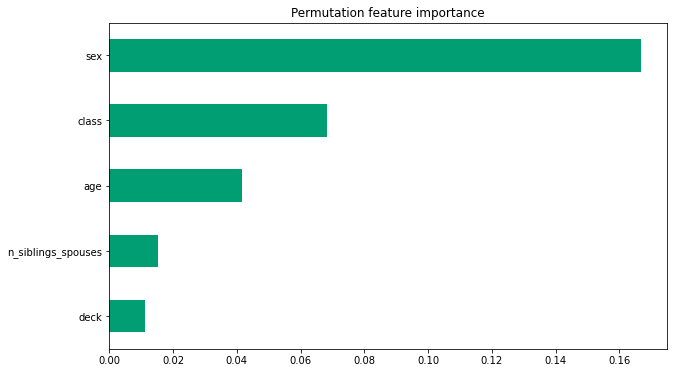## Visualizing model fitting

Lets first simulate/create training data using the following formula:

$$z=x* e^{-x^2 - y^2}$$

Where (z) is the dependent variable you are trying to predict and (x) and (y) are the features.

from numpy.random import uniform, seed
from scipy.interpolate import griddata

# Create fake data
seed(0)
npts = 5000
x = uniform(-2, 2, npts)
y = uniform(-2, 2, npts)
z = x*np.exp(-x**2 - y**2)
xy = np.zeros((2,np.size(x)))
xy = x
xy = y
xy = xy.T

# Prep data for training.
df = pd.DataFrame({'x': x, 'y': y, 'z': z})

xi = np.linspace(-2.0, 2.0, 200),
yi = np.linspace(-2.1, 2.1, 210),
xi,yi = np.meshgrid(xi, yi)

df_predict = pd.DataFrame({
'x' : xi.flatten(),
'y' : yi.flatten(),
})
predict_shape = xi.shape

def plot_contour(x, y, z, **kwargs):
# Grid the data.
plt.figure(figsize=(10, 8))
# Contour the gridded data, plotting dots at the nonuniform data points.
CS = plt.contour(x, y, z, 15, linewidths=0.5, colors='k')
CS = plt.contourf(x, y, z, 15,
vmax=abs(zi).max(), vmin=-abs(zi).max(), cmap='RdBu_r')
plt.colorbar()  # Draw colorbar.
# Plot data points.
plt.xlim(-2, 2)
plt.ylim(-2, 2)


You can visualize the function. Redder colors correspond to larger function values.

zi = griddata(xy, z, (xi, yi), method='linear', fill_value='0')
plot_contour(xi, yi, zi)
plt.scatter(df.x, df.y, marker='.')
plt.title('Contour on training data')
plt.show()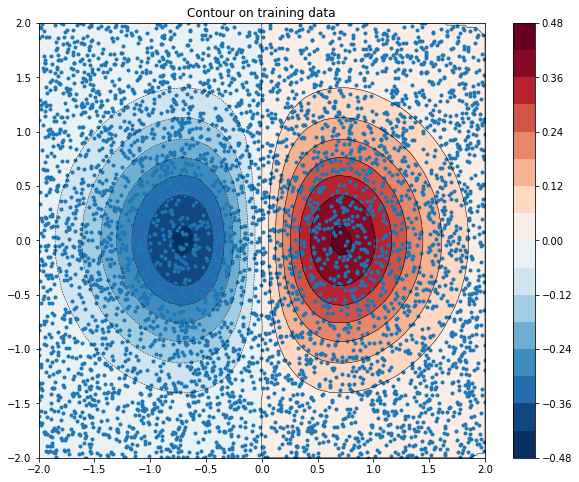fc = [tf.feature_column.numeric_column('x'),
tf.feature_column.numeric_column('y')]

def predict(est):
"""Predictions from a given estimator."""
predict_input_fn = lambda: tf.data.Dataset.from_tensors(dict(df_predict))
preds = np.array([p['predictions'] for p in est.predict(predict_input_fn)])
return preds.reshape(predict_shape)


First let's try to fit a linear model to the data.

train_input_fn = make_input_fn(df, df.z)
est = tf.estimator.LinearRegressor(fc)
est.train(train_input_fn, max_steps=500);

INFO:tensorflow:Using default config.
WARNING:tensorflow:Using temporary folder as model directory: /tmp/tmpd4fqobc9
INFO:tensorflow:Using config: {'_model_dir': '/tmp/tmpd4fqobc9', '_tf_random_seed': None, '_save_summary_steps': 100, '_save_checkpoints_steps': None, '_save_checkpoints_secs': 600, '_session_config': allow_soft_placement: true
graph_options {
rewrite_options {
meta_optimizer_iterations: ONE
}
}
, '_keep_checkpoint_max': 5, '_keep_checkpoint_every_n_hours': 10000, '_log_step_count_steps': 100, '_train_distribute': None, '_device_fn': None, '_protocol': None, '_eval_distribute': None, '_experimental_distribute': None, '_experimental_max_worker_delay_secs': None, '_session_creation_timeout_secs': 7200, '_service': None, '_cluster_spec': ClusterSpec({}), '_task_type': 'worker', '_task_id': 0, '_global_id_in_cluster': 0, '_master': '', '_evaluation_master': '', '_is_chief': True, '_num_ps_replicas': 0, '_num_worker_replicas': 1}
INFO:tensorflow:Calling model_fn.
WARNING:tensorflow:From /tensorflow-2.1.0/python3.6/tensorflow_core/python/feature_column/feature_column_v2.py:518: Layer.add_variable (from tensorflow.python.keras.engine.base_layer) is deprecated and will be removed in a future version.
Instructions for updating:
Please use layer.add_weight method instead.
WARNING:tensorflow:From /tensorflow-2.1.0/python3.6/tensorflow_core/python/keras/optimizer_v2/ftrl.py:143: calling Constant.__init__ (from tensorflow.python.ops.init_ops) with dtype is deprecated and will be removed in a future version.
Instructions for updating:
Call initializer instance with the dtype argument instead of passing it to the constructor
INFO:tensorflow:Done calling model_fn.
INFO:tensorflow:Create CheckpointSaverHook.
INFO:tensorflow:Graph was finalized.
INFO:tensorflow:Running local_init_op.
INFO:tensorflow:Done running local_init_op.
INFO:tensorflow:Saving checkpoints for 0 into /tmp/tmpd4fqobc9/model.ckpt.
INFO:tensorflow:loss = 0.023290718, step = 0
INFO:tensorflow:global_step/sec: 267.329
INFO:tensorflow:loss = 0.017512696, step = 100 (0.377 sec)
INFO:tensorflow:global_step/sec: 312.355
INFO:tensorflow:loss = 0.018098738, step = 200 (0.321 sec)
INFO:tensorflow:global_step/sec: 341.77
INFO:tensorflow:loss = 0.019927984, step = 300 (0.291 sec)
INFO:tensorflow:global_step/sec: 307.825
INFO:tensorflow:loss = 0.01797011, step = 400 (0.327 sec)
INFO:tensorflow:Saving checkpoints for 500 into /tmp/tmpd4fqobc9/model.ckpt.
INFO:tensorflow:Loss for final step: 0.019703189.


plot_contour(xi, yi, predict(est))

INFO:tensorflow:Calling model_fn.
WARNING:tensorflow:Layer linear/linear_model is casting an input tensor from dtype float64 to the layer's dtype of float32, which is new behavior in TensorFlow 2.  The layer has dtype float32 because it's dtype defaults to floatx.

If you intended to run this layer in float32, you can safely ignore this warning. If in doubt, this warning is likely only an issue if you are porting a TensorFlow 1.X model to TensorFlow 2.

To change all layers to have dtype float64 by default, call tf.keras.backend.set_floatx('float64'). To change just this layer, pass dtype='float64' to the layer constructor. If you are the author of this layer, you can disable autocasting by passing autocast=False to the base Layer constructor.

INFO:tensorflow:Done calling model_fn.
INFO:tensorflow:Graph was finalized.
INFO:tensorflow:Restoring parameters from /tmp/tmpd4fqobc9/model.ckpt-500
INFO:tensorflow:Running local_init_op.
INFO:tensorflow:Done running local_init_op.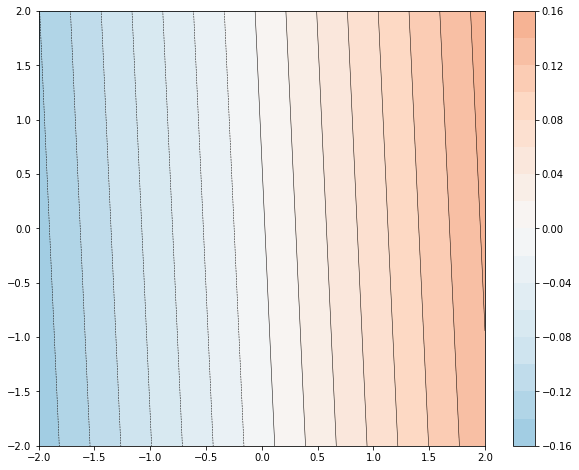It's not a very good fit. Next let's try to fit a GBDT model to it and try to understand how the model fits the function.

n_trees = 37

est = tf.estimator.BoostedTreesRegressor(fc, n_batches_per_layer=1, n_trees=n_trees)
est.train(train_input_fn, max_steps=500)
clear_output()
plot_contour(xi, yi, predict(est))
plt.text(-1.8, 2.1, '# trees: {}'.format(n_trees), color='w', backgroundcolor='black', size=20)
plt.show()

INFO:tensorflow:Calling model_fn.
INFO:tensorflow:Done calling model_fn.
INFO:tensorflow:Graph was finalized.
INFO:tensorflow:Restoring parameters from /tmp/tmp3jae7fgc/model.ckpt-222
INFO:tensorflow:Running local_init_op.
INFO:tensorflow:Done running local_init_op.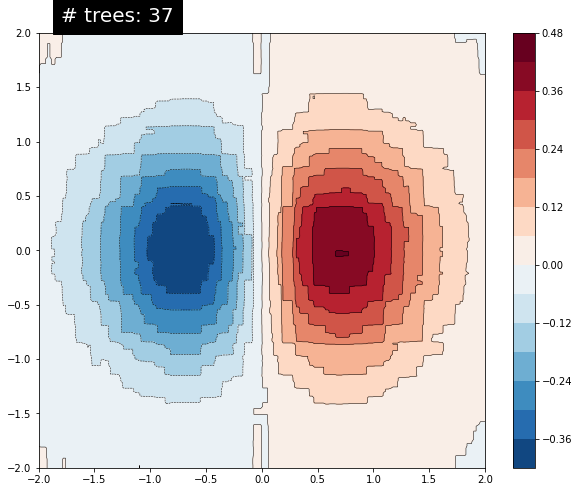As you increase the number of trees, the model's predictions better approximates the underlying function.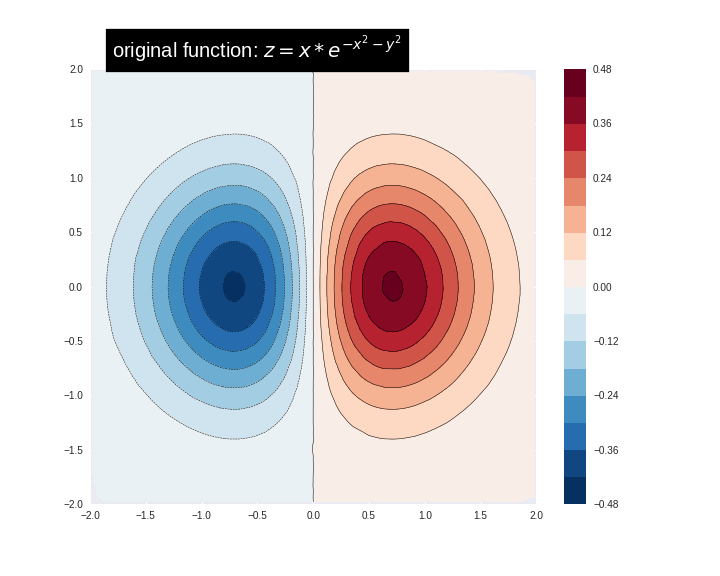## Conclusion

In this tutorial you learned how to interpret Boosted Trees models using directional feature contributions and feature importance techniques. These techniques provide insight into how the features impact a model's predictions. Finally, you also gained intution for how a Boosted Tree model fits a complex function by viewing the decision surface for several models.